# Analytics Calculations: Group and Summarize Results

Moderator, Lightspeed Staff Posts: 87 moderator
Warning, this Discussion involves breaking some conventions of our best practices, and will rely upon custom names that you assign to Calculations. If you use different naming conventions during the steps below, the raw code listed will not work as expected. However, the logic of the process should be the same.

Sometimes we can build in-table summaries of the results that Analytics gives us.

Let's say that we want to summarize results by only the subcategory, or using the top-level and secondary category together. We could build Calculations onto Analytics to build sums and gather them together in a meaningful way.

Let's say that we started with the First Subcategory report that we customized earlier, where we start with the complete category, but then remove everything following the subcategory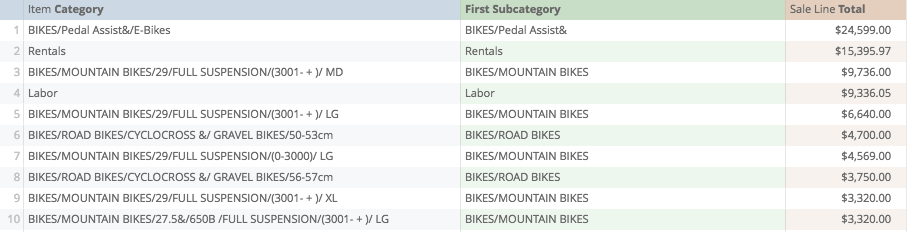We could prepare data to gather totals by First Subcategory, but we would need Calculations to do this, as we cannot use a calculated field as a dimension.

These are the logical steps we need to make this happen:

• Sort the results by First Subcategory
• Determine which is the last instance of each First Subcategory
• Count How many rows in which the same First Subcategory can be found
• Gather the results from each collection of First Subcategories
• Gather the First Subcategories and their respective results.

A bit of a note, the value of some of these steps may not be immediately clear, however, each piece is contributing to a meaningful completed product. I welcome any recommendations though on ways to improve this process.

The crux calculation that we are preparing is Offset List, one of the tools from our Table Functions

## Step OneSort by First Subcategory

This should be easy enough, we can do this by just clicking on the "Item Category" label, and this should sort everything accordingly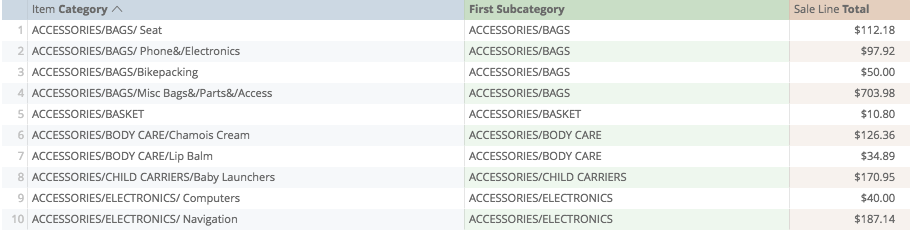So far so good

## Step TwoIs this First Subcategory the same as the next one?

This will help us identify the last instance of every First Subcategory.

Let's start now using a simple Offset function. I'm going to use Offset to look at the "First Subcategory" field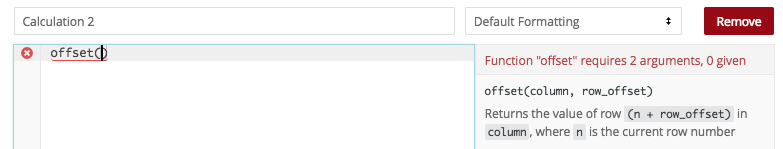and to ask it to look one row down...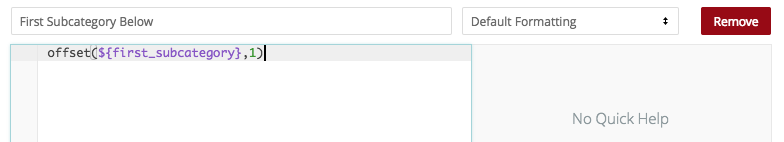so when I save, to the right of each First Subcategory, we should see the next First Subcategory...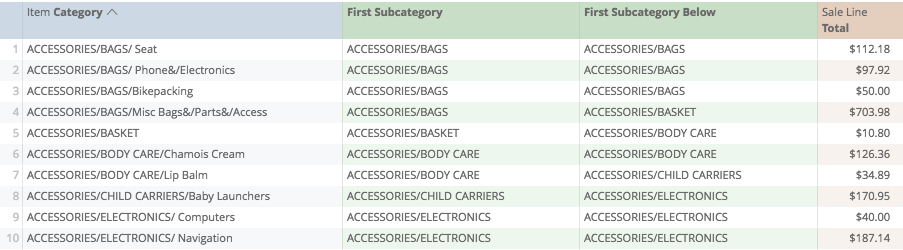Then, what we want to see if they are the same. We can do this with one more simple calculation: =

If the values are equal, Analytics will return a Yes, if not, Analytics will return a No.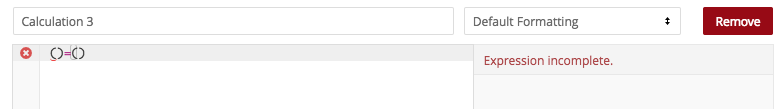...look for the "First Subcategory" calculation...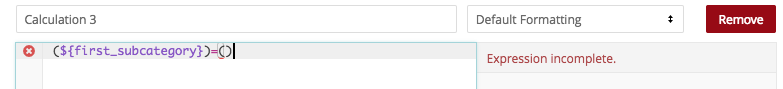...copy the offset function looking at the row below...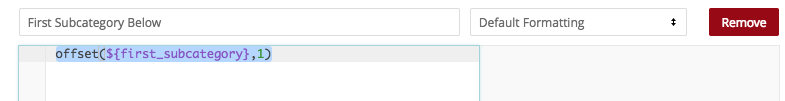...and then paste it into the second set of brackets...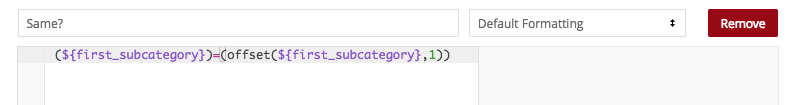...so that when we save...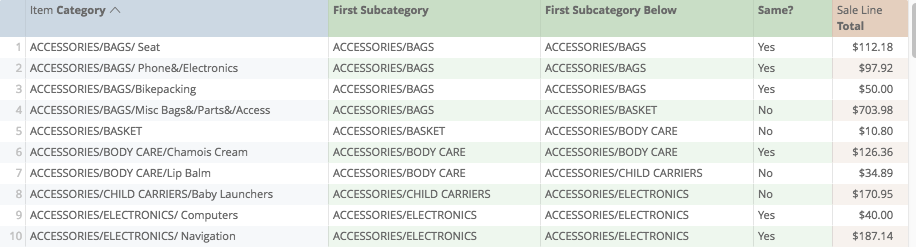We get a Yes/No calculation where the "No" identifies the final instance of every First Subcategory.

Let's save our work so far as new...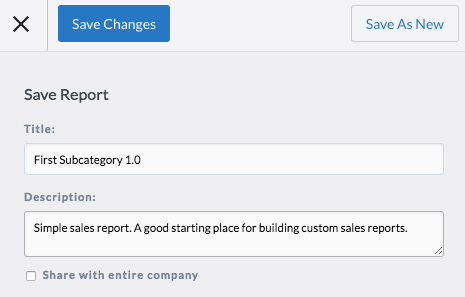## Step ThreeHow many rows are the same?

So now we know the last instance of each First Subcategory, but we also will need a count of each one.

A way to start this involves the Match function. Match returns the first row on which a value occurs.

What we will do is match the First Subcategory...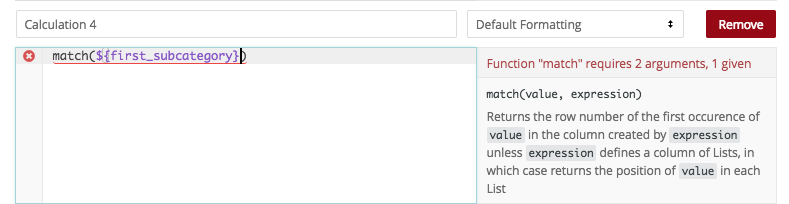...to the first subcategory...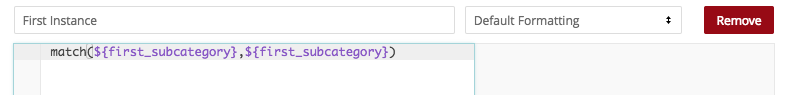...so when I save, we will get a Calculation repeating the first row on which each First Subcategory occurred...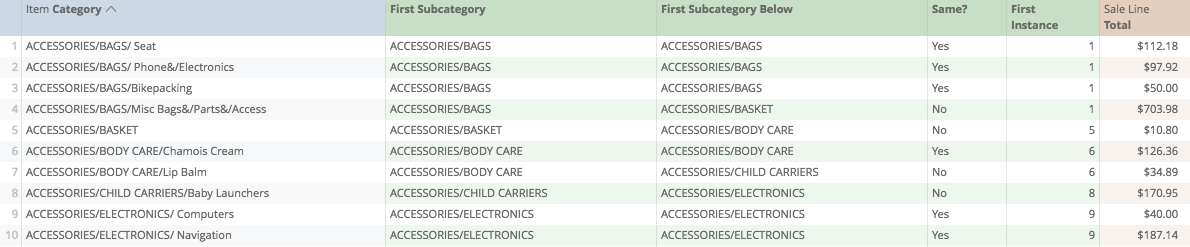Can you guess now how we can count the number of rows each First Category occupies?

Well, now we'll start a Calculation where we return the row()...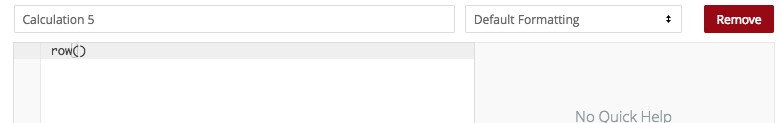...and then subtract...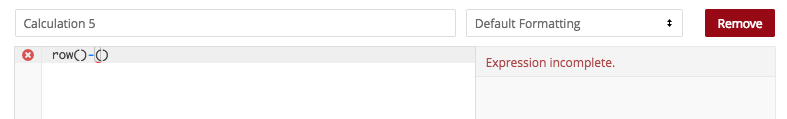..the matched reference...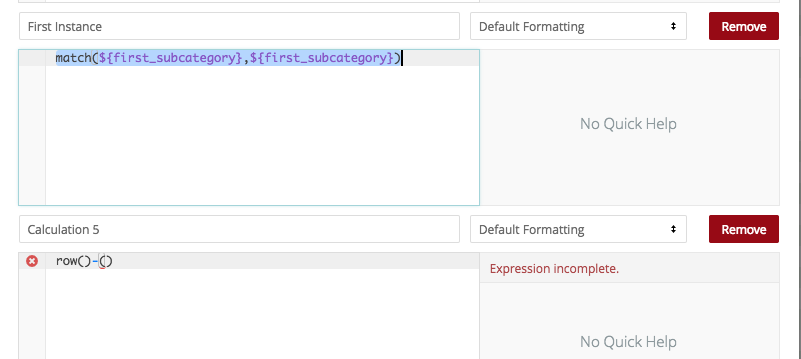...so that our calculation will look like...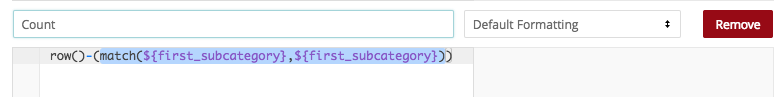...upon saving...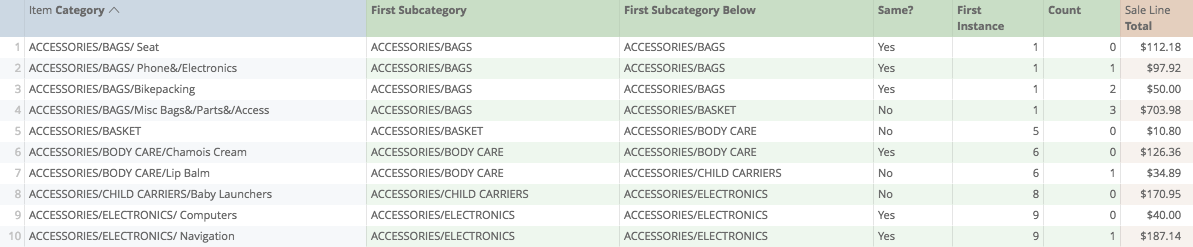...we will get a 0 for each First instance of a First Subcategory, and a subsequent count on each row of the subcategory.

Now let's save our changes...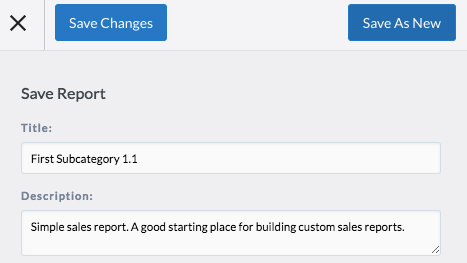## Step FourCreate a first and last reference point

The Offset List function we're going to use will need three arguments,

• the values (which will be our Sales Line totals)
• our start point for counting (or, how far back we want to start)
• the end point for counting (or, how many rows we wish to include)

Our values are already on the table.

Our start point now will be the negative row count for each last instance of a First Subcategory. So this becomes a pretty simple IF statement.

If we're looking at the last instance of the First Subcategory, show me the negative count, if not, return a 0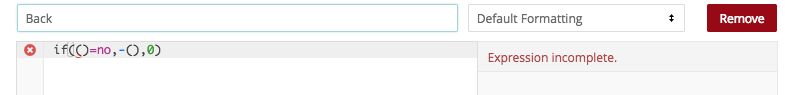...then I'm going to copy the calculation looking at the reference being the same or not...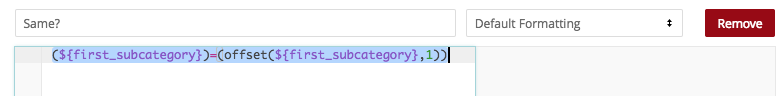...and paste, then grab the second function looking at the Count, copying...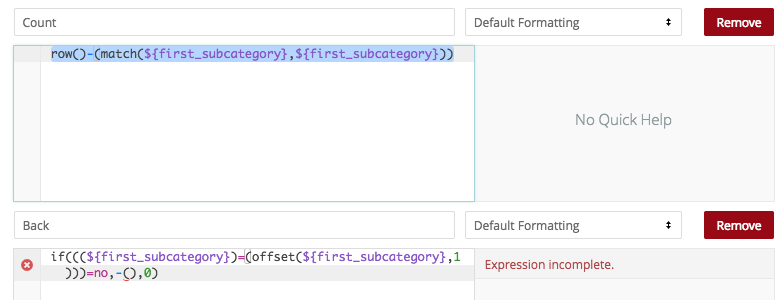...and pasting...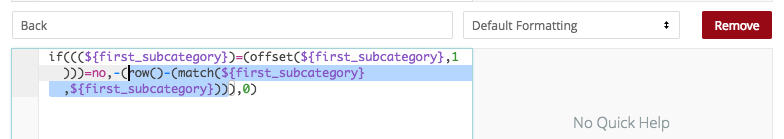...now when we save, for each "No", we should see the negative total, for each "Yes" we should see zero...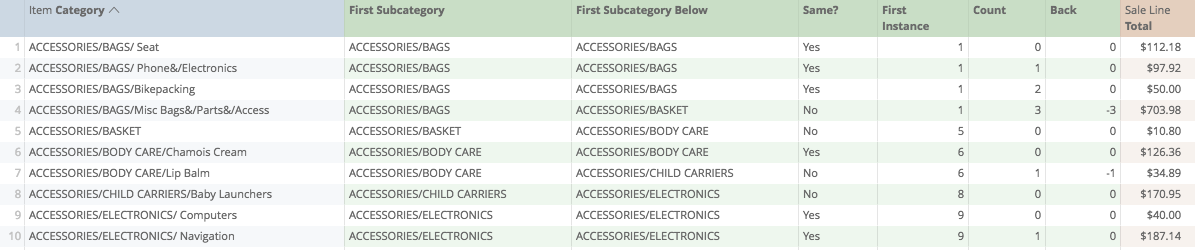Now, we want to define the end point. So this will need to be the row count plus one. Why you say? Because each count starts with zero.

We can copy+paste the same basic function above...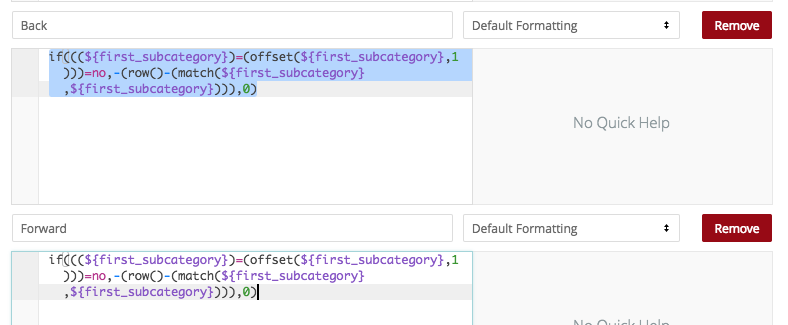but substitute the negative sign...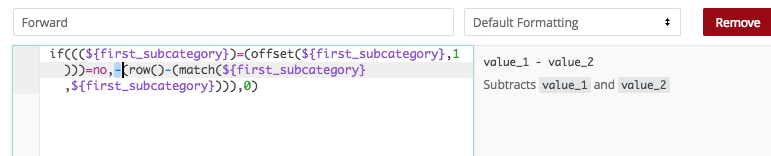...with a positive 1...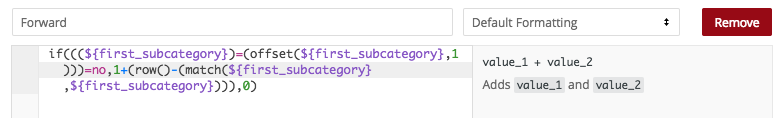...so upon saving...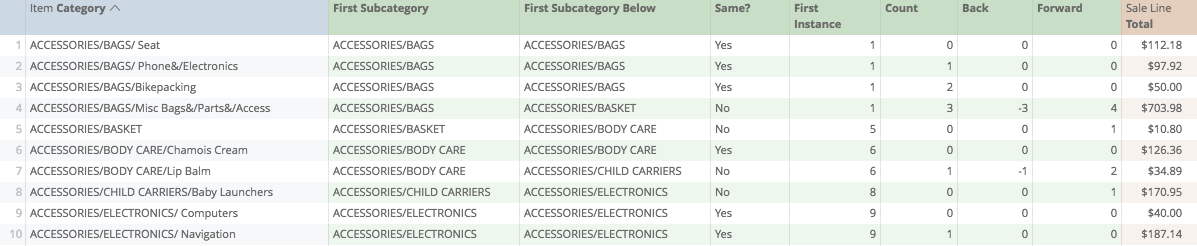We get yet another calculation with the opposite inverse number plus one...

Let's save our work...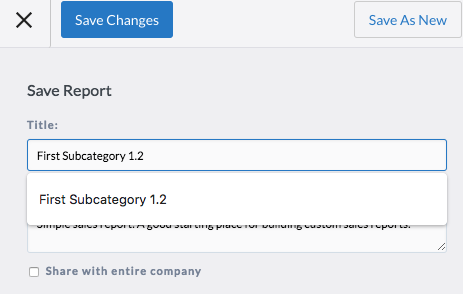## Step FiveGather the results

In case you were wondering. This is where the fun starts.

Now we get to roll out the offset_list function that we mentioned above.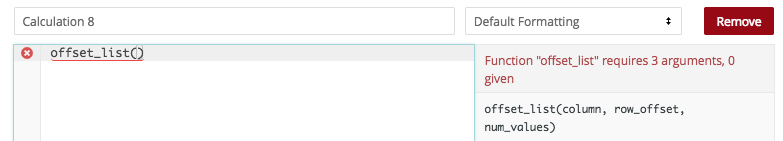First, what value are we looking at? I'm going to reference the sales totals...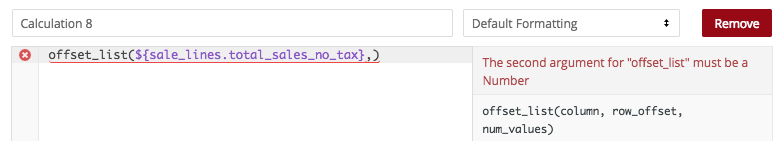...starting with our "Back" Calculation...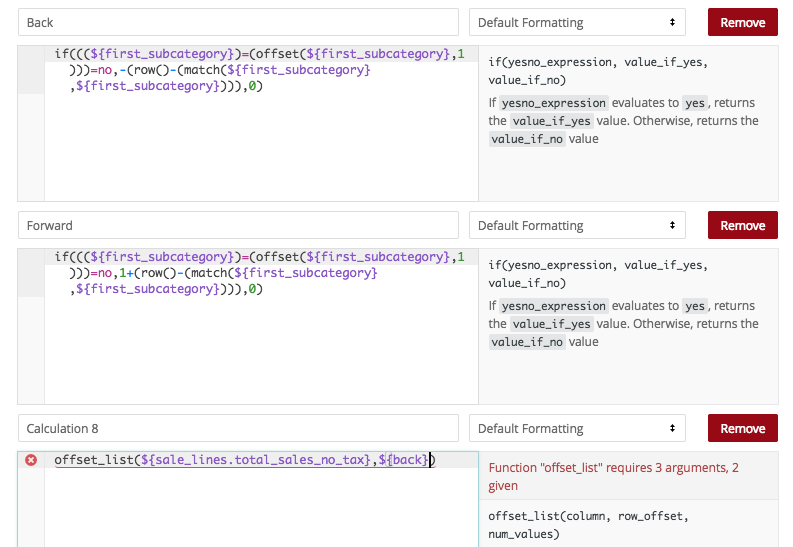...ending with our "Forward" Calculation...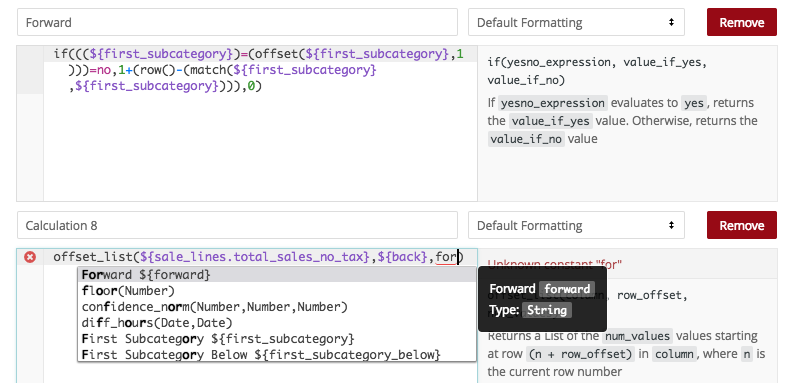...so our final calculation looks like...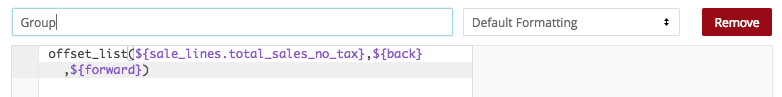...and when we save...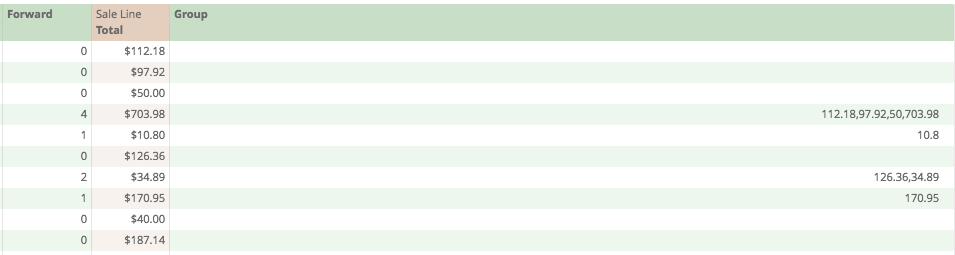wayyyyy over to the right, we'll see a list of all the Sale Line totals. Each list should only be displayed on the last instance of each First Subcategory, and should reference only its respective totals...

Now, one more step, let's create a sum...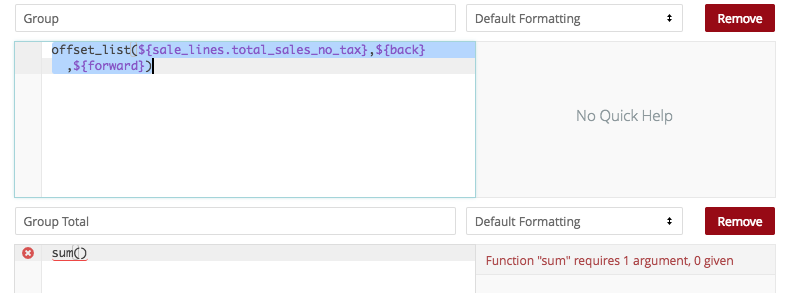...of that group total...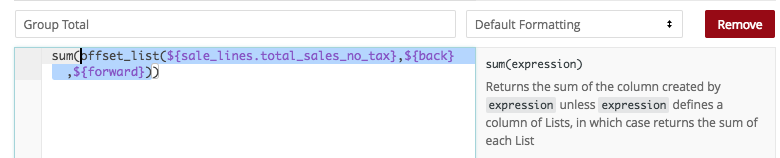...so that when we save, where applicable, we get one total per First Subcategory...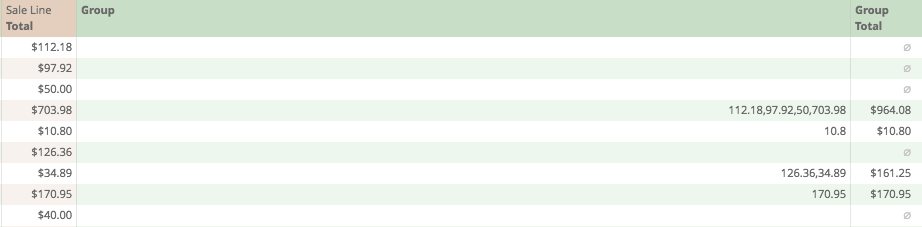Now let's save our work to this step...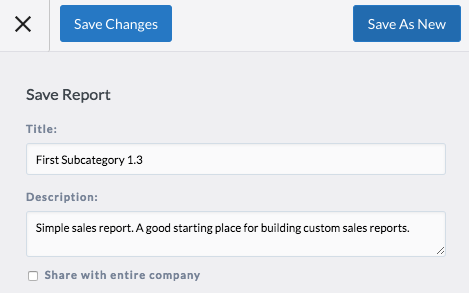## Step SixA bit of cleanup

So there are some supportive calculations here that we shouldn't need on this report. We are using First Subcategory, Back, Forward, and Group Total, we're also going to use "Same" at least once or twice more. Everything else we should be good to drop...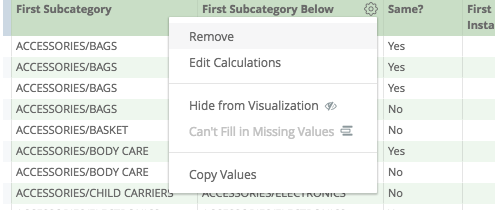So our lighter report to this point should look a bit like...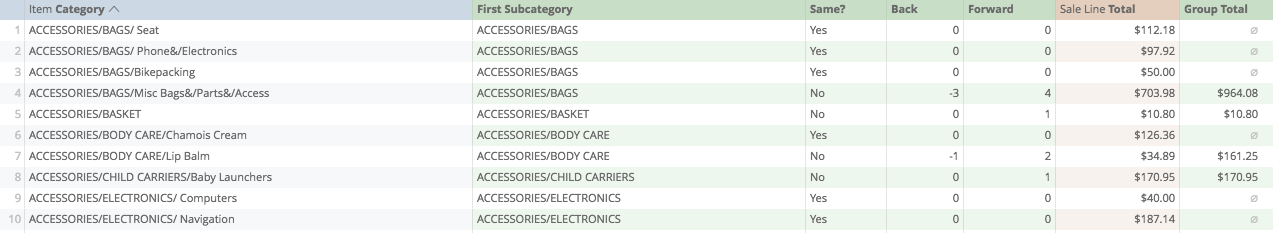## Step SevenLook for the Last Results

So now, we want to prime our report for gathering the meaningful data.

To start, I'm going to ask Analytics to return a 1 for every instance where "Same" is No, and return 0 otherwise.

So we'll start our calculation with brackets...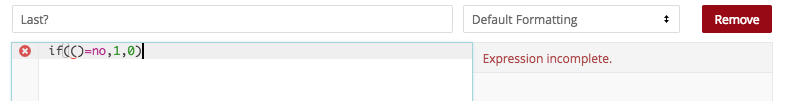and then copy+paste our calculation checking whether the First Subcategory is the same as the one below...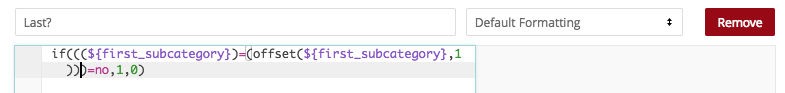When I save this, we should see a 0 for each Yes, and a 1 for each No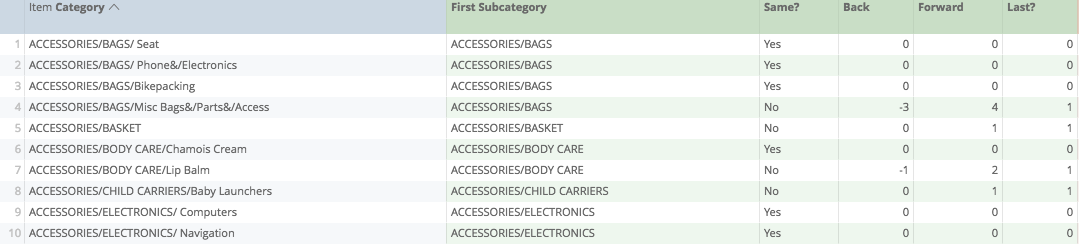Now what I want to do, is have these ones rise in sequence. How do we do that? Let's use our running_total function to return that number...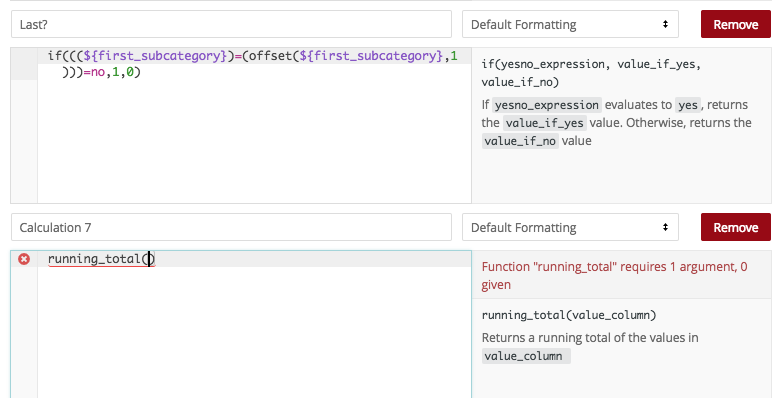copying...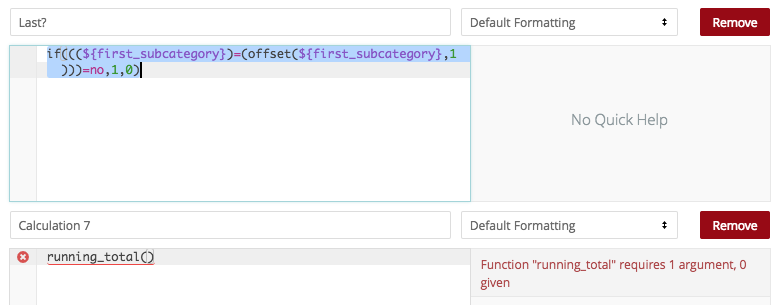...pasting...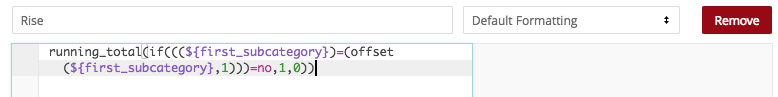...and saving...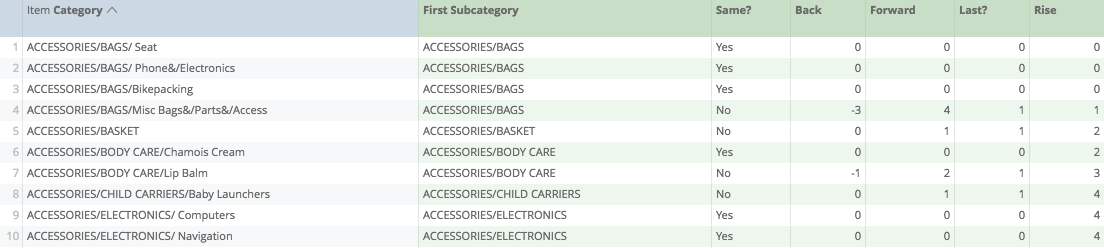now the next fun part, we are going to match the row of the report...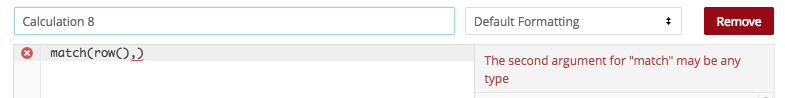...to this rising number...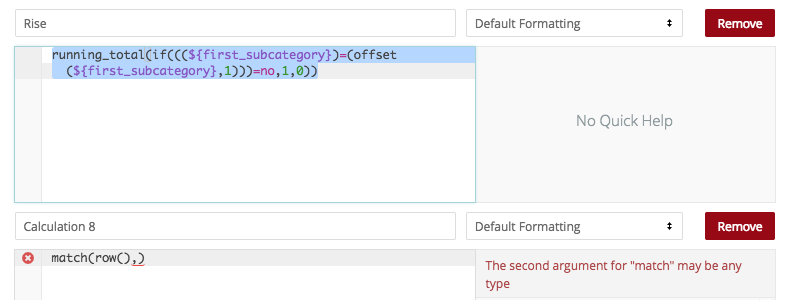...pasting...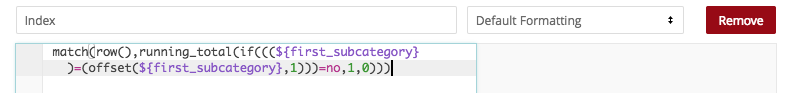...so that when we save...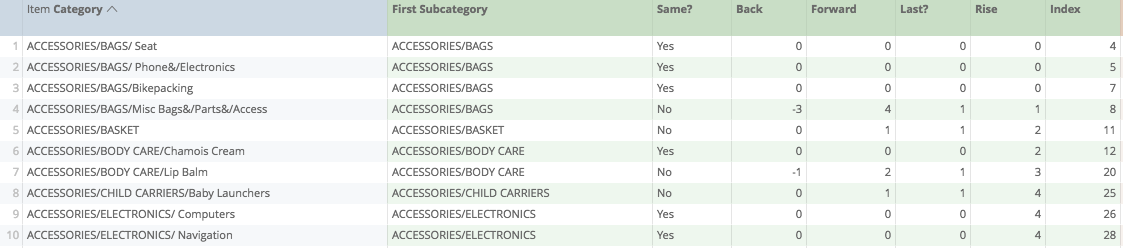Each row will return where the next First Subcategory can be found

See where we're going with this yet? We're almost there.

Let's save.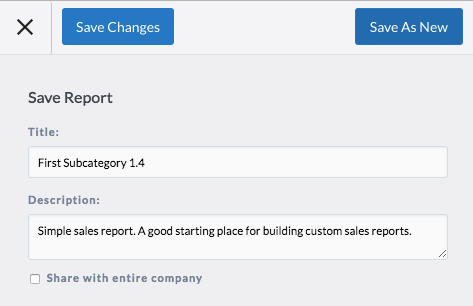## Step EightGather Labels and Totals

Now, to complete the preparation of our data, we're going to use the Index function...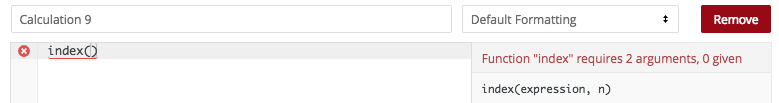To look at the First Subcategory labels that we created...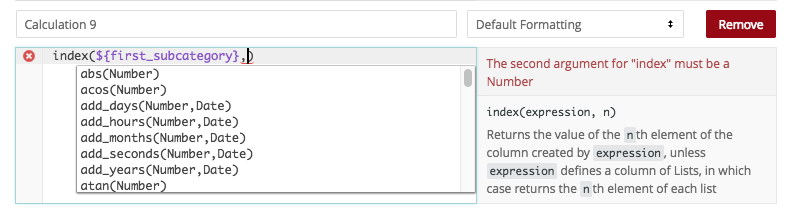...then from them, gather the indexed number...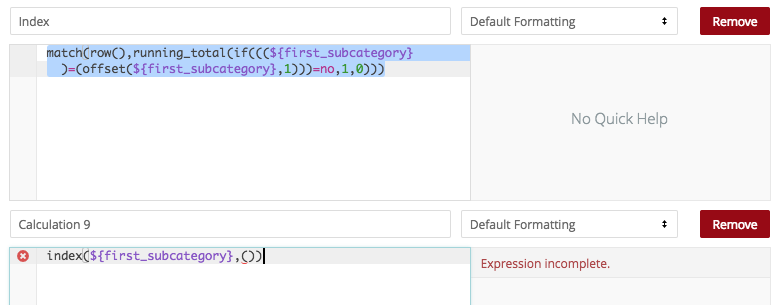copying, pasting...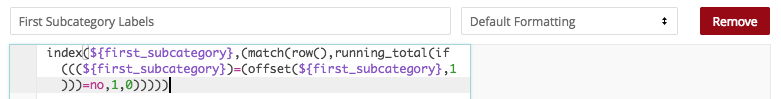...and saving...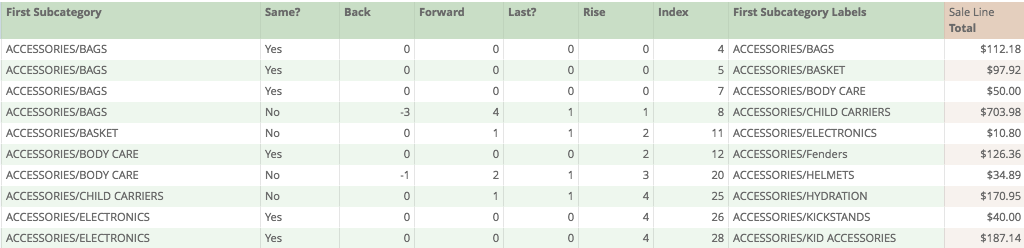creating a singular entry for all our First Subcategories...

finally, let's do the same for the grouped totals we created in Step Five...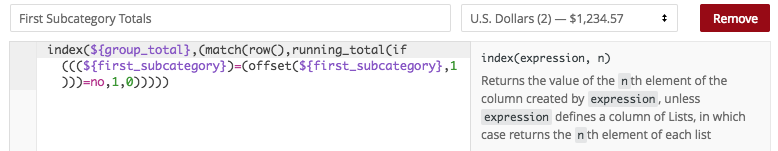so that when we save...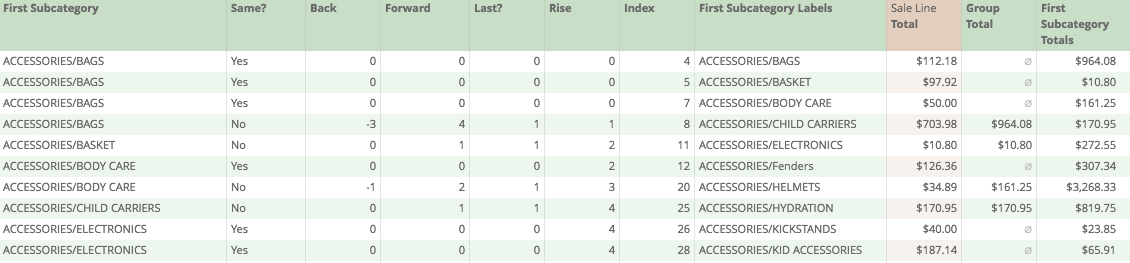We get...

well, we get a messy table of unclear numbers.

We're almost there. I promise.

## Step NineHide the Raw Data

If we open the Table Visualization now, we should see all the starter data...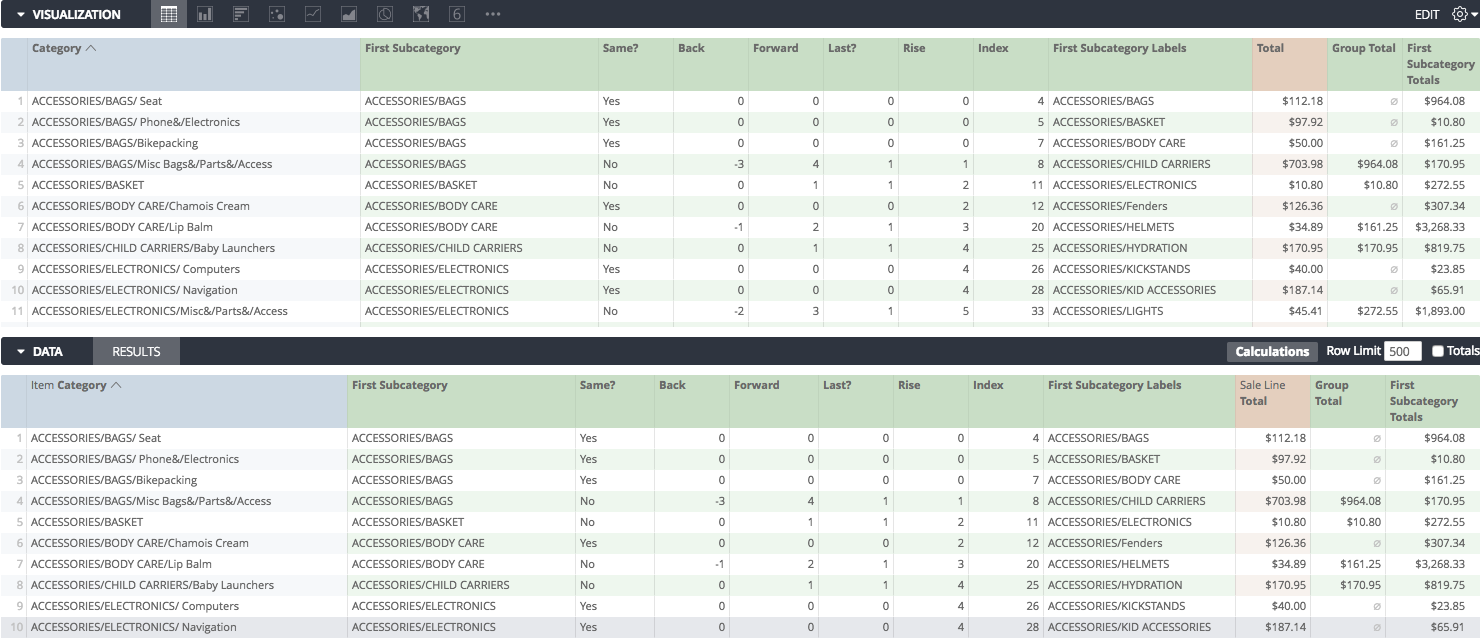However, only two of these columns are now of any interest to us...

So let's start hiding everything...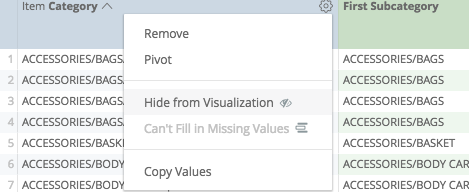...except...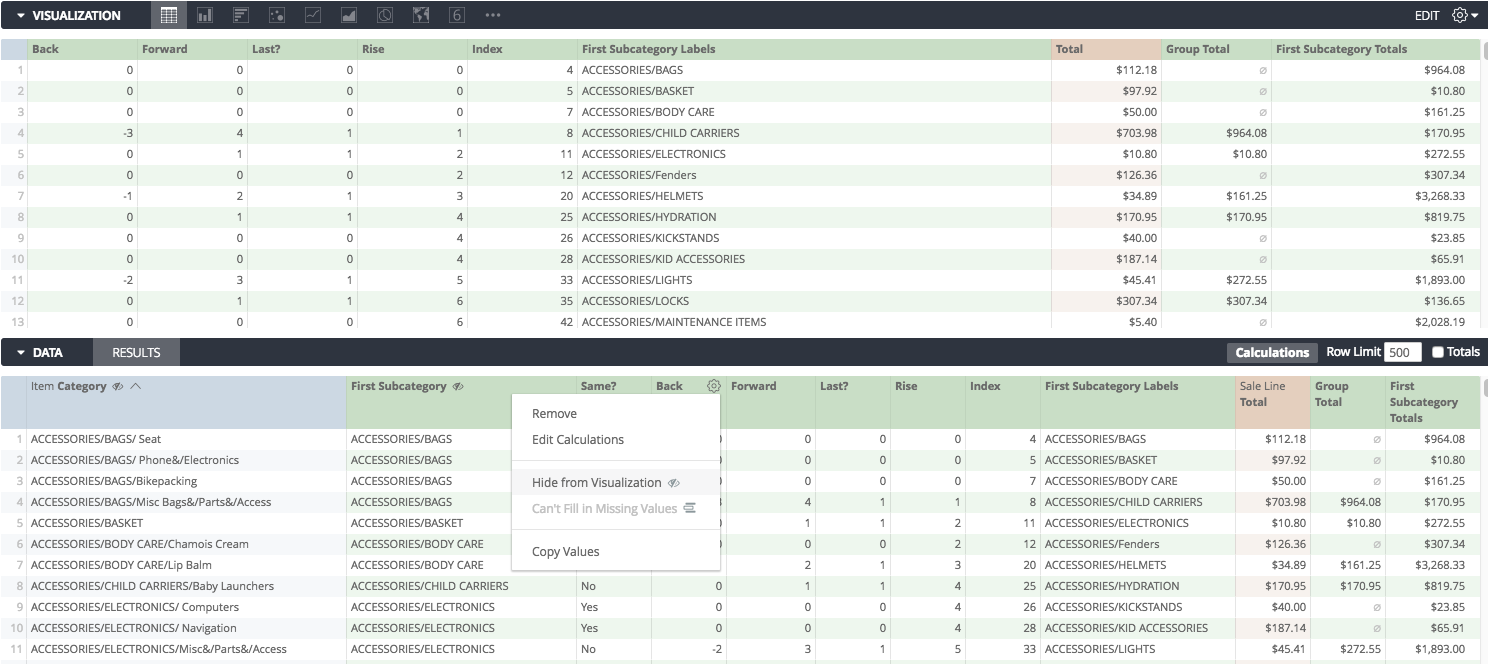...our labels...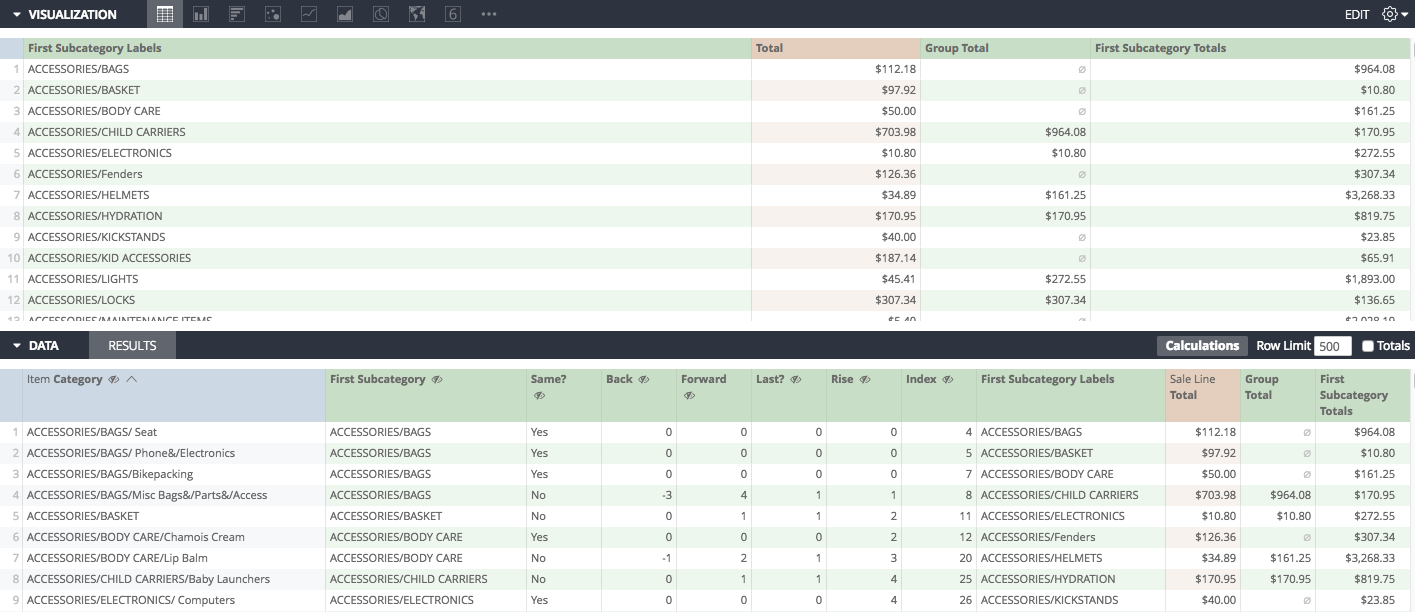...and the grouped totals...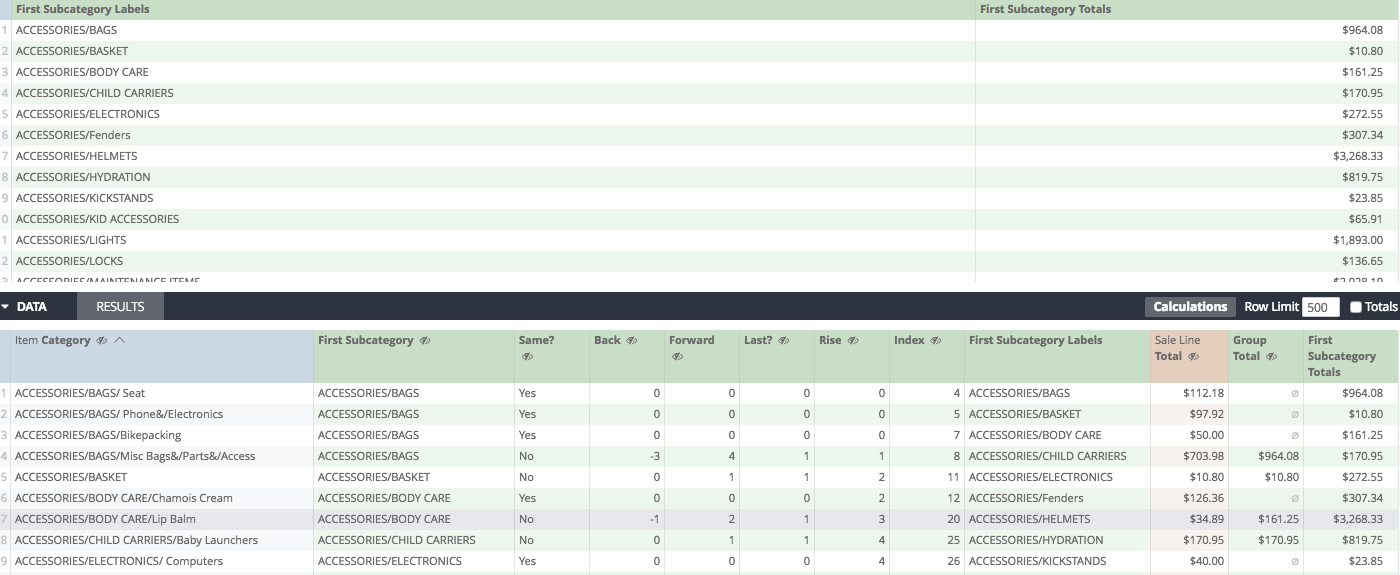So now, when we look at the Visualization...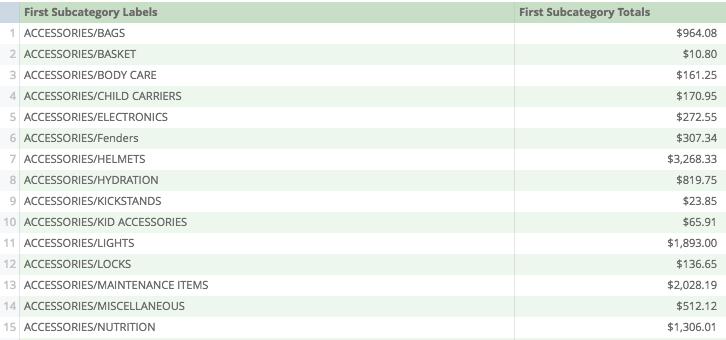We get a clean list of all the summary totals by First Subcategory!

Gosh, if you made it this far, you're awesome. Treat yourself to something nice.

Calculations Home
Joseph McKeown
Lightspeed Analytics and reporting consultant
Lightspeed HQ